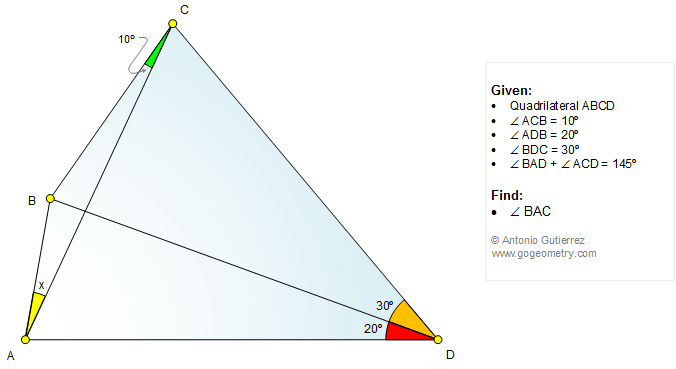Geometry Problem 1152: Triangle, Quadrilateral, Angle, 10, 20, 30 Degrees. Level: School, College, Mathematics Education

< PREVIOUS PROBLEM  |  NEXT PROBLEM >

 The figure below shows a quadrilateral ABCD, angle ACB = 10 degrees, angle ADB = 20 degrees, angle BDC = 30 degrees, and angle BAD + angle ACD =145. Find angle BAC.This entry contributed by Sumith Peiris, Moratuwa, Sri Lanka.See also: Triangles Quadrilaterals Angles Post or view a solution

 Selected Topics

 Home | Search | Geometry | Problems | All Problems | Open Problems | Visual Index | 10 Problems | Problems Art Gallery | Art | 1151-1160 | Triangles | Quadrilaterals | Angles | Last updated: Oct 10 2015